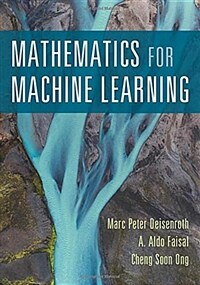> Detail View

# Detail View## Mathematics for machine learning (Loan 5 times)

Material type
단행본
Personal Author
Deisenroth, Marc Peter, author. Faisal, A. Aldo, author. Ong, Cheng Soon, author.
Title Statement
Mathematics for machine learning / Marc Peter Deisenroth, A. Aldo Faisal, Cheng Soon Ong.
Publication, Distribution, etc
Cambridge, UK ;   New York, NY :   Cambridge University Press,   2020.
Physical Medium
xvii, 371 p. : ill. (some col.) ; 26 cm.
ISBN
9781108470049 (hardcover) 1108470041 (hardcover) 9781108455145 (paperback) 110845514X (paperback) 9781108679930 (electronic publication)
요약
"The fundamental mathematical tools needed to understand machine learning include linear algebra, analytic geometry, matrix decompositions, vector calculus, optimization, probability, and statistics. These topics are traditionally taught in disparate courses, making it hard for data science or computer science students, or professionals, to efficiently learn the mathematics. This self-contained textbook bridges the gap between mathematical and machine learning texts, introducing the mathematical concepts with a minimum of prerequisites. It uses these concepts to derive four central machine learning methods: linear regression, principal component analysis, Gaussian mixture models, and support vector machines. For students and others with a mathematical background, these derivations provide a starting point to machine learning texts. For those learning the mathematics for the first time, the methods help build intuition and practical experience with applying mathematical concepts"--
Content Notes
Introduction and motivation -- Linear algebra -- Analytic geometry -- Matrix decompositions -- Vector calculus -- Probability and distribution -- Continuous optimization -- When models meet data -- Linear regression -- Dimensionality reduction with principal component analysis -- Density estimation with Gaussian mixture models -- Classification with support vector machines.
Bibliography, Etc. Note
Includes bibliographical references (p. 357-366) and index.
Machine learning --Mathematics.
 000 00000cam u2200205 a 4500 001 000046097368 005 20211105094303 008 211104s2020 enka b 001 0 eng 010 ▼a 2019040762 015 ▼a GBC022925 ▼2 bnb 020 ▼a 9781108470049 (hardcover) 020 ▼a 1108470041 (hardcover) 020 ▼a 9781108455145 (paperback) 020 ▼a 110845514X (paperback) 020 ▼a 9781108679930 (electronic publication) 035 ▼a (KERIS)REF000019428058 040 ▼a LBSOR/DLC ▼b eng ▼e rda ▼c DLC ▼d OCLCO ▼d UKMGB ▼d YDX ▼d 211009 042 ▼a pcc 050 0 0 ▼a Q325.5 ▼b .D45 2020 082 0 0 ▼a 006.3/1 ▼2 23 084 ▼a 006.31 ▼2 DDCK 090 ▼a 006.31 ▼b D325m 100 1 ▼a Deisenroth, Marc Peter, ▼e author. 245 1 0 ▼a Mathematics for machine learning / ▼c Marc Peter Deisenroth, A. Aldo Faisal, Cheng Soon Ong. 260 ▼a Cambridge, UK ; ▼a New York, NY : ▼b Cambridge University Press, ▼c 2020. 264 1 ▼a Cambridge, United Kingdom ; ▼a New York, NY : ▼b Cambridge University Press, ▼c 2020. 300 ▼a xvii, 371 p. : ▼b ill. (some col.) ; ▼c 26 cm. 336 ▼a text ▼b txt ▼2 rdacontent 337 ▼a unmediated ▼b n ▼2 rdamedia 338 ▼a volume ▼b nc ▼2 rdacarrier 504 ▼a Includes bibliographical references (p. 357-366) and index. 505 0 ▼a Introduction and motivation -- Linear algebra -- Analytic geometry -- Matrix decompositions -- Vector calculus -- Probability and distribution -- Continuous optimization -- When models meet data -- Linear regression -- Dimensionality reduction with principal component analysis -- Density estimation with Gaussian mixture models -- Classification with support vector machines. 520 ▼a "The fundamental mathematical tools needed to understand machine learning include linear algebra, analytic geometry, matrix decompositions, vector calculus, optimization, probability, and statistics. These topics are traditionally taught in disparate courses, making it hard for data science or computer science students, or professionals, to efficiently learn the mathematics. This self-contained textbook bridges the gap between mathematical and machine learning texts, introducing the mathematical concepts with a minimum of prerequisites. It uses these concepts to derive four central machine learning methods: linear regression, principal component analysis, Gaussian mixture models, and support vector machines. For students and others with a mathematical background, these derivations provide a starting point to machine learning texts. For those learning the mathematics for the first time, the methods help build intuition and practical experience with applying mathematical concepts"-- ▼c Provided by publisher. 650 0 ▼a Machine learning ▼x Mathematics. 700 1 ▼a Faisal, A. Aldo, ▼e author. 700 1 ▼a Ong, Cheng Soon, ▼e author. 945 ▼a KLPA

### Holdings Information

No. Location Call Number Accession No. Availability Due Date Make a Reservation Service
No. 1 Location Call Number 006.31 D325m Accession No. 121258371 Availability Available Due Date Make a Reservation Service

### Contents information

`1. Introduction and motivation; 2. Linear algebra; 3. Analytic geometry; 4. Matrix decompositions; 5. Vector calculus; 6. Probability and distribution; 7. Optimization; 8. When models meet data; 9. Linear regression; 10. Dimensionality reduction with principal component analysis; 11. Density estimation with Gaussian mixture models; 12. Classification with support vector machines.`

### New Arrivals Books in Related Fields

김성민 (2023)

반병현 (2022)

#### 딥러닝을 위한 수학

Kneusel, Ronald T (2022)

이병준 (2022)

#### 컴퓨터 비전 : 원리, 알고리듬, 응용

Davies, E. R (2022)

추상목 (2022)

정원섭 (2022)

심수진 (2022)

#### 실무자를 위한 딥러닝

Kneusel, Ronald T (2022)

김화종 (2022)

#### 비즈니스를 위한 AI : 머신러닝과 인공신경망에 알아야 할 모든 것 / 제2판

Rose, Doug (2022)

배정철 (2022)

김종완 (2022)

한국교육학술정보원 (2022)

#### 데이터 민주화와 셀프서비스 데이터 : 모두가 쉽고 빠르게 데이터 인사이트를 도출하는 지름길

Uttamchandani, Sandeep (2022)

강우규 (2022)